• PyTorch全连接ReLU网络 1．PyTorch的核心是两个主要特征： • 一个n维张量，类似于numpy，但可以在GPU上运行 • 搭建和训练神经网络时的自动微分/求导机制 本文将使用全连接的ReLU网络作为运行示例。该网络将有一个...
PyTorch全连接ReLU网络 1．PyTorch的核心是两个主要特征： • 一个n维张量，类似于numpy，但可以在GPU上运行 • 搭建和训练神经网络时的自动微分/求导机制 本文将使用全连接的ReLU网络作为运行示例。该网络将有一个单一的隐藏层，并将使用梯度下降训练，通过最小化网络输出和真正结果的欧几里得距离，来拟合随机生成的数据。 2.张量 2.1 热身: Numpy 在介绍PyTorch之前，将首先使用numpy实现网络。 Numpy提供了一个n维数组对象，以及许多用于操作这些数组的函数。Numpy是用于科学计算的通用框架; 对计算图、深度学习和梯度一无所知。然而，可以很容易地使用NumPy，手动实现网络的前向和反向传播，来拟合随机数据：
-- coding: utf-8 --
import numpy as np
N是批量大小; D_in是输入维度;
49/5000 H是隐藏的维度; D_out是输出维度。
N, D_in, H, D_out = 64, 1000, 100, 10
创建随机输入和输出数据
x = np.random.randn(N, D_in) y = np.random.randn(N, D_out)
随机初始化权重
w1 = np.random.randn(D_in, H) w2 = np.random.randn(H, D_out)
learning_rate = 1e-6 for t in range(500): # 前向传递：计算预测值y h = x.dot(w1) h_relu = np.maximum(h, 0) y_pred = h_relu.dot(w2)
# 计算和打印损失loss
loss = np.square(y_pred - y).sum()
print(t, loss)

# 反向传播，计算w1和w2对loss的梯度
grad_y_pred = 2.0 * (y_pred - y)

# 更新权重

2.2 PyTorch：张量 Numpy是一个很棒的框架，但不能利用GPU来加速其数值计算。 对于现代深度神经网络，GPU通常提供50倍或更高的加速，所以，numpy不能满足当代深度学习的需求。 在这里，先介绍最基本的PyTorch概念： 张量（Tensor）：PyTorch的tensor在概念上与numpy的array相同： tensor是一个n维数组，PyTorch提供了许多函数，用于操作这些张量。任何希望使用NumPy执行的计算，也可以使用PyTorch的tensor来完成，可以认为是科学计算的通用工具。 与Numpy不同，PyTorch可以利用GPU加速其数值计算。要在GPU上运行Tensor, 在构造张量使用device参数把tensor建立在GPU上。 本文使用tensors将随机数据上训练一个两层的网络。和前面NumPy的例子类似，使用PyTorch的tensor，手动在网络中实现前向传播和反向传播：
-- coding: utf-8 --
import torch
dtype = torch.float device = torch.device(“cpu”)
device = torch.device（“cuda：0”）＃取消注释以在GPU上运行
N是批量大小; D_in是输入维度;
H是隐藏的维度; D_out是输出维度。
N, D_in, H, D_out = 64, 1000, 100, 10
#创建随机输入和输出数据 x = torch.randn(N, D_in, device=device, dtype=dtype) y = torch.randn(N, D_out, device=device, dtype=dtype)
随机初始化权重
w1 = torch.randn(D_in, H, device=device, dtype=dtype) w2 = torch.randn(H, D_out, device=device, dtype=dtype)
learning_rate = 1e-6 for t in range(500): # 前向传递：计算预测y h = x.mm(w1) h_relu = h.clamp(min=0) y_pred = h_relu.mm(w2)
# 计算和打印损失
loss = (y_pred - y).pow(2).sum().item()
print(t, loss)

# Backprop计算w1和w2相对于损耗的梯度
grad_y_pred = 2.0 * (y_pred - y)

# 使用梯度下降更新权重

-- coding: utf-8 --
import torch
dtype = torch.float device = torch.device(“cpu”)
device = torch.device（“cuda：0”）＃取消注释以在GPU上运行
N是批量大小; D_in是输入维度;
H是隐藏的维度; D_out是输出维度。
N, D_in, H, D_out = 64, 1000, 100, 10
创建随机Tensors以保持输入和输出。
在向后传球期间对于这些Tensors。
x = torch.randn(N, D_in, device=device, dtype=dtype) y = torch.randn(N, D_out, device=device, dtype=dtype)
为权重创建随机Tensors。
在向后传球期间尊重这些张贴。
w1 = torch.randn(D_in, H, device=device, dtype=dtype, requires_grad=True) w2 = torch.randn(H, D_out, device=device, dtype=dtype, requires_grad=True)
learning_rate = 1e-6 for t in range(500): # 前向传播：使用tensors上的操作计算预测值y; # 由于w1和w2有requires_grad=True，涉及这些张量的操作将让PyTorch构建计算图， # 从而允许自动计算梯度。由于不再手工实现反向传播，所以不需要保留中间值的引用。 y_pred = x.mm(w1).clamp(min=0).mm(w2)
# 使用Tensors上的操作计算和打印丢失。
# loss是一个形状为()的张量
# loss.item() 得到这个张量对应的python数值
loss = (y_pred - y).pow(2).sum()
print(t, loss.item())

loss.backward()

# 使用梯度下降更新权重。对于这一步，只想对w1和w2的值进行原地改变；不想为更新阶段构建计算图，

# 反向传播后手动将梯度设置为零

3.2 PyTorch：定义新的自动求导函数 在底层，每一个原始的自动求导运算，实际上是两个在Tensor上运行的函数。其中，forward函数计算，从输入Tensors获得的输出Tensors。而backward函数接收输出Tensors，对于某个标量值的梯度，并且计算输入Tensors相对于该相同标量值的梯度。 在PyTorch中，可以很容易地通过定义torch.autograd.Function的子类，实现forward和backward函数，来定义自己的自动求导运算。之后，就可以使用这个新的自动梯度运算符了。然后，可以通过构造一个实例并像调用函数一样，传入包含输入数据的tensor调用，这样来使用新的自动求导运算。 这个例子中，自定义一个自动求导函数，来展示ReLU的非线性。并用实现的两层网络： import torch
class MyReLU(torch.autograd.Function): “”" 可以通过建立torch.autograd的子类来实现自定义的autograd函数， 并完成张量的正向和反向传播。 “”" @staticmethod def forward(ctx, x): “”" 在正向传播中，接收到一个上下文对象和一个包含输入的张量； 必须返回一个包含输出的张量， 并且可以使用上下文对象来缓存对象，以便在反向传播中使用。 “”" ctx.save_for_backward(x) return x.clamp(min=0)
@staticmethod
"""
在反向传播中，接收到上下文对象和一个张量，
其包含了相对于正向传播过程中产生的输出的损失的梯度。
可以从上下文对象中检索缓存的数据，
并且必须计算并返回与正向传播的输入相关的损失的梯度。
"""
x, = ctx.saved_tensors

device = torch.device(‘cuda’ if torch.cuda.is_available() else ‘cpu’)
N是批大小； D_in 是输入维度；
H 是隐藏层维度； D_out 是输出维度
N, D_in, H, D_out = 64, 1000, 100, 10
产生输入和输出的随机张量
x = torch.randn(N, D_in, device=device) y = torch.randn(N, D_out, device=device)
产生随机权重的张量
w1 = torch.randn(D_in, H, device=device, requires_grad=True) w2 = torch.randn(H, D_out, device=device, requires_grad=True)
learning_rate = 1e-6 for t in range(500): # 正向传播：使用张量上的操作来计算输出值y； # 通过调用 MyReLU.apply 函数来使用自定义的ReLU y_pred = MyReLU.apply(x.mm(w1)).mm(w2)
# 计算并输出loss
loss = (y_pred - y).pow(2).sum()
print(t, loss.item())

loss.backward()

# 用梯度下降更新权重

# 在反向传播之后手动清零梯度

3.3 TensorFlow：静态图 PyTorch自动求导看起来非常像TensorFlow：这两个框架中，都定义计算图，使用自动微分来计算梯度。两者最大的不同就是TensorFlow的计算图是静态的，而PyTorch使用动态的计算图。 在TensorFlow中，定义计算图一次，然后重复执行这个相同的图，可能会提供不同的输入数据。而在PyTorch中，每一个前向通道定义一个新的计算图。 静态图的好处在于，可以预先对图进行优化。例如，一个框架可能要融合一些图的运算来提升效率，或者产生一个策略来将图分布到多个GPU或机器上。如果重复使用相同的图，那么在重复运行同一个图时，前期潜在的代价高昂的预先优化的消耗就会被分摊开。 静态图和动态图的一个区别是控制流。对于一些模型，希望对每个数据点执行不同的计算。例如，一个递归神经网络，可能对于每个数据点，执行不同的时间步数，这个展开（unrolling）可以作为一个循环来实现。对于一个静态图，循环结构要作为图的一部分。因此，TensorFlow提供了运算符（例如tf.scan）来把循环嵌入到图当中。对于动态图来说，情况更加简单：既然为每个例子即时创建图，可以使用普通的命令式控制流，来为每个输入执行不同的计算。 为了与上面的PyTorch自动梯度实例做对比，使用TensorFlow来拟合一个简单的2层网络： import tensorflow as tf import numpy as np
首先建立计算图（computational graph）
N是批大小；D是输入维度；
H是隐藏层维度；D_out是输出维度。
N, D_in, H, D_out = 64, 1000, 100, 10
为输入和目标数据创建placeholder；
当执行计算图时，他将会被真实的数据填充
x = tf.placeholder(tf.float32, shape=(None, D_in)) y = tf.placeholder(tf.float32, shape=(None, D_out))
为权重创建Variable并用随机数据初始化
TensorFlow的Variable在执行计算图时不会改变
w1 = tf.Variable(tf.random_normal((D_in, H))) w2 = tf.Variable(tf.random_normal((H, D_out)))
前向传播：使用TensorFlow的张量运算计算预测值y。
注意这段代码实际上不执行任何数值运算；
只是建立了稍后将执行的计算图。
h = tf.matmul(x, w1) h_relu = tf.maximum(h, tf.zeros(1)) y_pred = tf.matmul(h_relu, w2)
使用TensorFlow的张量运算损失（loss）
loss = tf.reduce_sum((y - y_pred) ** 2.0)
计算loss对于w1和w2的导数
使用梯度下降更新权重。为了实际更新权重，需要在执行计算图时计算new_w1和new_w2。
注意，在TensorFlow中，更新权重值的行为是计算图的一部分;
但在PyTorch中，这发生在计算图形之外。
learning_rate = 1e-6 new_w1 = w1.assign(w1 - learning_rate * grad_w1) new_w2 = w2.assign(w2 - learning_rate * grad_w2)
现在搭建好了计算图，所以开始一个TensorFlow的会话（session）来实际执行计算图。
with tf.Session() as sess:
# 运行一次计算图来初始化Variable w1和w2
sess.run(tf.global_variables_initializer())

# 创建numpy数组来存储输入x和目标y的实际数据
x_value = np.random.randn(N, D_in)
y_value = np.random.randn(N, D_out)

for _ in range(500):
# 多次运行计算图。每次执行时，都用feed_dict参数，
# 将x_value绑定到x，将y_value绑定到y，
# 每次执行图形时都要计算损失、new_w1和new_w2；
# 这些张量的值以numpy数组的形式返回。
loss_value, _, _ = sess.run([loss, new_w1, new_w2],
feed_dict={x: x_value, y: y_value})
print(loss_value)

-- coding: utf-8 --
import torch
N是批大小；D是输入维度
H是隐藏层维度；D_out是输出维度
N, D_in, H, D_out = 64, 1000, 100, 10
#创建输入和输出随机张量 x = torch.randn(N, D_in) y = torch.randn(N, D_out)
使用nn包将的模型定义为一系列的层。
nn.Sequential是包含其他模块的模块，并按顺序应用这些模块来产生其输出。
每个线性模块使用线性函数从输入计算输出，并保存其内部的权重和偏差张量。
在构造模型之后，使用.to()方法将其移动到所需的设备。
model = torch.nn.Sequential( torch.nn.Linear(D_in, H), torch.nn.ReLU(), torch.nn.Linear(H, D_out), )
nn包还包含常用的损失函数的定义；
在这种情况下，将使用平均平方误差(MSE)作为的损失函数。
设置reduction=‘sum’，表示计算的是平方误差的“和”，而不是平均值;
这是为了与前面手工计算损失的例子保持一致，
但是在实践中，通过设置reduction='elementwise_mean’来使用均方误差作为损失更为常见。
loss_fn = torch.nn.MSELoss(reduction=‘sum’)
learning_rate = 1e-4 for t in range(500): # 前向传播：通过向模型传入x计算预测的y。 # 模块对象重载了__call__运算符，所以可以像函数那样调用。 # 这么做相当于向模块传入了一个张量，然后返回了一个输出张量。 y_pred = model(x)
 # 计算并打印损失。
# 传递包含y的预测值和真实值的张量，损失函数返回包含损失的张量。
loss = loss_fn(y_pred, y)
print(t, loss.item())

# 反向传播之前清零梯度

# 反向传播：计算模型的损失对所有可学习参数的导数（梯度）。
# 因此这个调用将计算模型中所有可学习参数的梯度。
loss.backward()

# 使用梯度下降更新权重。
# 每个参数都是张量，所以可以像以前那样可以得到的数值和梯度
for param in model.parameters():

N是批大小；D是输入维度
H是隐藏层维度；D_out是输出维度
N, D_in, H, D_out = 64, 1000, 100, 10
产生随机输入和输出张量
x = torch.randn(N, D_in) y = torch.randn(N, D_out)
使用nn包定义模型和损失函数
model = torch.nn.Sequential( torch.nn.Linear(D_in, H), torch.nn.ReLU(), torch.nn.Linear(H, D_out), ) loss_fn = torch.nn.MSELoss(reduction=‘sum’)
使用optim包定义优化器（Optimizer）。Optimizer将会为更新模型的权重。
learning_rate = 1e-4 optimizer = torch.optim.Adam(model.parameters(), lr=learning_rate)
for t in range(500):
# 前向传播：通过像模型输入x计算预测的y
y_pred = model(x)

# 计算并打印loss
loss = loss_fn(y_pred, y)
print(t, loss.item())

# 在反向传播之前，使用optimizer将要更新的所有张量的梯度清零(这些张量是模型可学习的权重)

# 反向传播：根据模型的参数计算loss的梯度
loss.backward()

# 调用Optimizer的step函数使所有参数更新
optimizer.step()

4.3 PyTorch：自定义nn模块 有时候，需要指定比现有模块序列更复杂的模型；对于这些情况，可以通过继承nn.Module并定义forward函数，这个forward函数可以使用其他模块或者其他的自动求导运算，来接收输入tensor，产生输出tensor。 在这个例子中，用自定义Module的子类构建两层网络： import torch
class TwoLayerNet(torch.nn.Module): def init(self, D_in, H, D_out): “”" 在构造函数中，实例化了两个nn.Linear模块，并将作为成员变量。 “”" super(TwoLayerNet, self).init() self.linear1 = torch.nn.Linear(D_in, H) self.linear2 = torch.nn.Linear(H, D_out)
def forward(self, x):
"""
在前向传播的函数中，接收一个输入的张量，也必须返回一个输出张量。
可以使用构造函数中定义的模块以及张量上的任意的（可微分的）操作。
"""
h_relu = self.linear1(x).clamp(min=0)
y_pred = self.linear2(h_relu)
return y_pred

N是批大小； D_in 是输入维度；
H 是隐藏层维度； D_out 是输出维度
N, D_in, H, D_out = 64, 1000, 100, 10
产生输入和输出的随机张量
x = torch.randn(N, D_in) y = torch.randn(N, D_out)
通过实例化上面定义的类来构建的模型。
model = TwoLayerNet(D_in, H, D_out)
构造损失函数和优化器。
SGD构造函数中对model.parameters()的调用，
将包含模型的一部分，即两个nn.Linear模块的可学习参数。
loss_fn = torch.nn.MSELoss(reduction=‘sum’) optimizer = torch.optim.SGD(model.parameters(), lr=1e-4) for t in range(500): # 前向传播：通过向模型传递x计算预测值y y_pred = model(x)
#计算并输出loss
loss = loss_fn(y_pred, y)
print(t, loss.item())

# 清零梯度，反向传播，更新权重
loss.backward()
optimizer.step()

4.4 PyTorch：控制流和权重共享 作为动态图和权重共享的一个例子，实现了一个非常奇怪的模型：一个全连接的ReLU网络，在每一次前向传播时，隐藏层的层数为随机1到4之间的数，这样可以多次重用相同的权重来计算。 因为这个模型可以使用普通的Python流控制来实现循环，并且可以通过在定义转发时，多次重用同一个模块，来实现最内层之间的权重共享。 利用Mudule的子类很容易实现这个模型： import random import torch
class DynamicNet(torch.nn.Module): def init(self, D_in, H, D_out): “”" 在构造函数中，构造了三个nn.Linear实例，将在前向传播时被使用。 “”" super(DynamicNet, self).init() self.input_linear = torch.nn.Linear(D_in, H) self.middle_linear = torch.nn.Linear(H, H) self.output_linear = torch.nn.Linear(H, D_out)
def forward(self, x):
"""
对于模型的前向传播，随机选择0、1、2、3，
并重用了多次计算隐藏层的middle_linear模块。
由于每个前向传播构建一个动态计算图，
可以在定义模型的前向传播时使用常规Python控制流运算符，如循环或条件语句。
在这里，还看到，在定义计算图形时多次重用同一个模块是完全安全的。
这是Lua Torch的一大改进，因为Lua Torch中每个模块只能使用一次。
"""
h_relu = self.input_linear(x).clamp(min=0)
for _ in range(random.randint(0, 3)):
h_relu = self.middle_linear(h_relu).clamp(min=0)
y_pred = self.output_linear(h_relu)
return y_pred

N是批大小；D是输入维度
H是隐藏层维度；D_out是输出维度
N, D_in, H, D_out = 64, 1000, 100, 10
产生输入和输出随机张量
x = torch.randn(N, D_in) y = torch.randn(N, D_out)
实例化上面定义的类来构造的模型
model = DynamicNet(D_in, H, D_out)
构造的损失函数（loss function）和优化器（Optimizer）。
用平凡的随机梯度下降训练这个奇怪的模型是困难的，所以使用了momentum方法。
criterion = torch.nn.MSELoss(reduction=‘sum’) optimizer = torch.optim.SGD(model.parameters(), lr=1e-4, momentum=0.9) for t in range(500):
# 前向传播：通过向模型传入x计算预测的y。
y_pred = model(x)

# 计算并打印损失
loss = criterion(y_pred, y)
print(t, loss.item())

# 清零梯度，反向传播，更新权重
loss.backward()
optimizer.step()

展开全文• 27_pytorch全连接层，使用MNIST的分类案例（学习笔记）.pdf
• nn.Linear relu? concisely inherit from nn.Module init layer in __init__ implement forward() step 1 step 2 step 3 nn.ReLU v.s. F.relu() class-style API 因此需要先实例化 ......
nn.Linear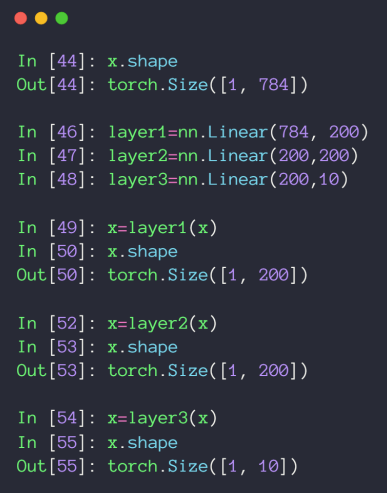relu?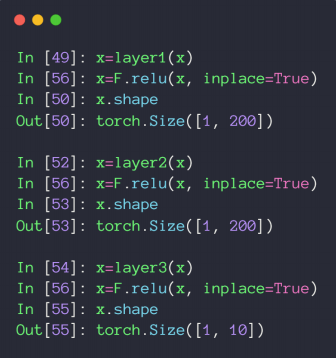concisely
inherit from nn.Moduleinit layer in __init__implement forward()
step 1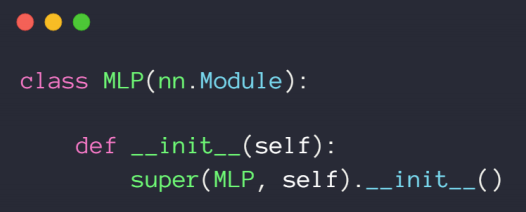step 2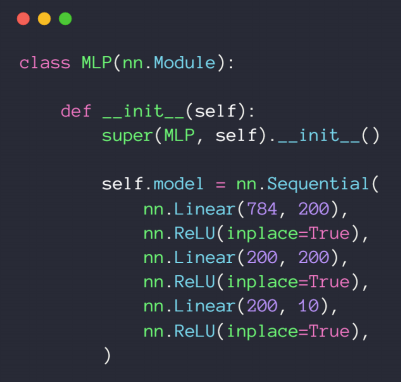step 3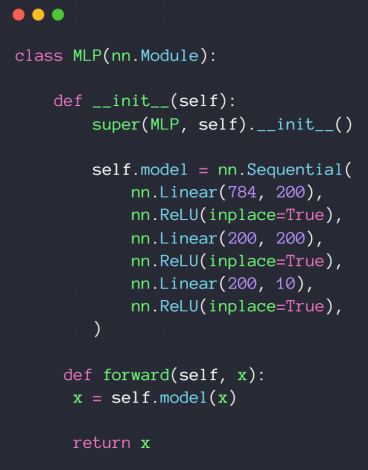nn.ReLU v.s. F.relu()
class-style API
因此需要先实例化function-style API
因此可以直接传参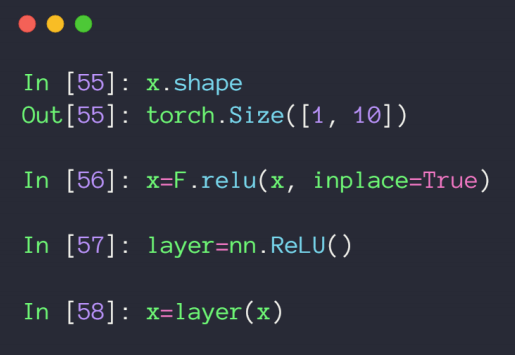Train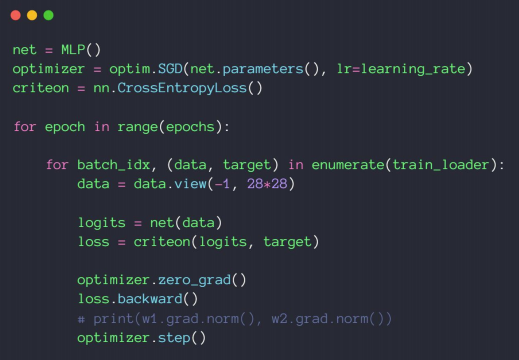展开全文深度学习 python
• ## Pytorch全连接网络

千次阅读 2019-11-29 19:55:34
后一部分构建了两种全连接网络，用三种不同方案拟合时序数据；并在例程中详细分析误差函数，优化器，网络调参，以及数据反向求导的过程。 数据预处理 本篇使用航空乘客数据AirPassengers.csv，其中包括从1949-1960...
本篇开始学习搭建真正的神经网络，前一部分讨论深度学习中预处理数据的基本流程；后一部分构建了两种全连接网络，用三种不同方案拟合时序数据；并在例程中详细分析误差函数，优化器，网络调参，以及数据反向求导的过程。
数据预处理
本篇使用航空乘客数据AirPassengers.csv，其中包括从1949-1960年每月旅客的数量，程序则用于预测未来几年中每月的旅客数量，数据可从以下Git项目中下载。
https://github.com/aarshayj/analytics_vidhya/blob/master/Articles/Time_Series_Analysis/AirPassengers.csv
1．读取数据
首先，引入必要的头文件，并从文件中读入数据：
import pandas as pd
import numpy as np
import matplotlib.pyplot as plt
%matplotlib inline

import torch
import torch.nn as nn

plt.plot(df['#Passengers'])
plt.show()

程序输出如下图所示：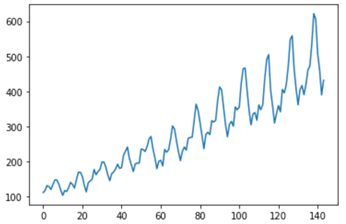2．归一化
无论机器学习还是深度学习，使用哪一种框架，归一化都是必要环节。归一化的目标是将每一维特征压缩到一定范围之内，以免不同特征因取值范围不同而影响其权重。非常大或非常小的值搭配上不恰当的学习率，往往使得收敛过慢，或者因每次调整的波动太大最终无法收敛。归一化去除了这些不稳定因素。
归一化的具体做法是将某一列特征转换成均值为 0、标准差为1的数据，在图像处理过程中，也常把0-255之间的颜色值转换为0-1之间的小数。
本例中使用了均值和标准差编写了归一化和反归一化函数：
def feature_normalize(data):
mu = np.mean(data,axis=0) # 均值
std = np.std(data,axis=0) # 标准差
return (data - mu)/std

def feature_unnormalize(data, arr):
mu = np.mean(data,axis=0)
std = np.std(data,axis=0)
return arr * std + mu

3．提取新特征
提取新特征是指从现有特征中提取更多可以代入模型的信息，从而生成新特征，本例中的数据包括两列，第一列“Month”是字符串类型的时间，第二列“#Passengers”是乘客量，也就是需要预测的数据y。下面通过拆分和类型转换，从第一列中提取具体的年“year”和月“mon”，将索引列变为特征“x”，并使用上面定义的函数实现归一化功能。
df['year'] = df['Month'].apply(lambda x: float(x[:4]))
df['mon'] = df['Month'].apply(lambda x: float(x[5:]))
df['x'] = feature_normalize(df.index)
df['y'] = feature_normalize(df['#Passengers'])
df['year'] = feature_normalize(df['year'])
df['mon'] = feature_normalize(df['mon'])
df['real'] = feature_unnormalize(df['#Passengers'], df['y'])

处理后的数据如下图所示：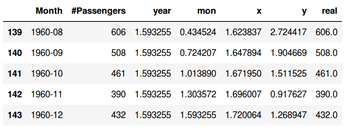4．处理缺失值和异常值
处理缺失值和异常值也是特征工程的重要环节，有时花费的时间比建模还多。处理缺失值的常用方法是删除重要特征缺失的item，或者用均值，前后值填充；处理异常值是监测数据中不正常的值，并做出相应处理，由于本例中数据比较“干净”，无需做缺失值和异常值处理。
5．向量化
向量化是将读出的数据转换成模型需要的数据格式，根据不同的模型做法不同，本例中的向量化将在后面的模型部分实现。
6．切分训练集和测试集
训练前还需要把数据切分成训练集和测试集，以避免过拟合，本例中将70%的数据用于训练，最终模型将对所有数据预测并做图。
TRAIN_PERCENT = 0.7
train_size = int(len(df) * TRAIN_PERCENT)
train = df[:train_size]

拟合直线
拟合程序分成三部分：定义模型、优化器和误差函数；训练模型；预测并做图。
1．定义模型、优化器、误差函数
模型继承自mm.Module，并实现了两个核心函数，init用于初始化模型结构，forward用于定义前向传播的过程。本例中实现了最为简单的模型，其中只包含一个全连接层，使用nn.Linear定义，torch.nn中定义了常用的网络层实现。
class LinearRegression(nn.Module):
def __init__(self):
super(LinearRegression, self).__init__()
self.linear = nn.Linear(1, 1) # 输入和输出的维度都是1

def forward(self, x):
x = self.linear(x)
return x

model = LinearRegression()
criterion = nn.MSELoss() # 损失函数：均方误差
optimizer = torch.optim.SGD(model.parameters(), lr=0.001) # 优化算法：随机梯度下降

损失函数使用了均方误差 MSELoss，它计算的是预测值与真值之差平方的期望值，MSELoss也是回归中最常用的损失函数，torch.nn中实现了一些常用的损失函数，可以直接使用，
优化的目标是更好地更新参数，使模型快速收敛。优化算法就是调整模型参数更新的策略，优化器是优化算法的具体实现。本例中优化器optimizer使用了最基础的随机梯度下降optim.SGD优化方法，torch.optim中定义了常用的优化器。在参数中设置了学习率为0.001，并将模型的参数句柄传入优化器，优化器后期将调整这些参数。
注意：学习率是一个重要参数，最好从小到大设置，如果设置太大，可能造成每次对参数修改过大，造成抖动，使得最终无法收敛。
2．训练模型
训练之前，先把数据转换成模型需要的数据格式，将pandas的数据格式转换为float32格式的Tensor张量，然后用unsqueeze扩展维度到2维（unsqueeze已在上一篇详细介绍）。
x = torch.unsqueeze(torch.tensor(np.array(train['x']), dtype=torch.float32), dim=1)
y = torch.unsqueeze(torch.tensor(np.array(train['y']), dtype=torch.float32), dim=1)

for e in range(10000):
inputs = Variable(x)
target = Variable(y)
out = model(inputs) # 前向传播
loss = criterion(out, target) # 计算误差
loss.backward() # 后向传播
optimizer.step() # 调整参数
if (e+1) % 1000 == 0: # 每1000次迭代打印一次误差值
print('Epoch:{}, Loss:{:.5f}'.format(e+1, loss.item()))

后面的循环部分进行了10000次迭代，也就是说将所有数据放进模型训练了10000次，从而使模型收敛。每一次循环之中，将x,y分别转换成变量Variable格式。
然后进行前先传播，model(inputs)调用的是nn.Module 的call()函数（call是Python类中的一个特殊方法，如果类中定义了此方法，可以通过实例名加括号的方式调用该方法）父类的call()调用了前向函数forward()将数据传入层中处理。
接下来是误差函数和优化器配合调整模型参数，此处到底修改了哪些值，又是如何修改的，是最难理解的部分。先通过定义的误差函数计算误差，从loss值可以看到每一次迭代之后误差的情况。
for p in model.parameters():

也可以在程序中加入以下代码，用于跟踪后向传播的过程：
f = loss.grad_fn
while True:
print(f)
if len(f.next_functions) == 0:
break
f = f.next_functions

3．预测和做图
本例中用70%数据作为训练集，用所有数据作为测试集，因此，用全部数据重新计算了x,y值；使用eval函数将模型转换为测试模式（有一些层在训练模型和预测模型时有差别）；将数据代入模型预测，并转换成numpy格式作图显示。
x = torch.unsqueeze(torch.tensor(np.array(df['x']), dtype=torch.float32), dim=1)
y = torch.unsqueeze(torch.tensor(np.array(df['y']), dtype=torch.float32), dim=1)
model.eval() #将模型变为测试模式
predict = model(Variable(x)) # 预测
predict = predict.data.numpy() # 转换成numpy格式
plt.plot(x.numpy(), y.numpy(), 'y')
plt.plot(x.numpy(), predict)
plt.show()

程序运行结果如下图所示，可以看到模型用一条直线拟合曲线，在前70%的训练数据中表现更好。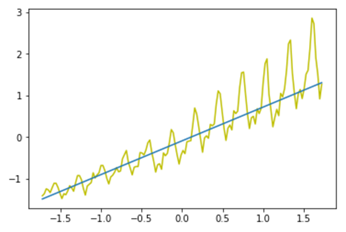多特征拟合
直线拟合的原理是y=kx+b，求斜率k和截距b。其中的x是数据产生的时间，从数据表的索引号转换求得，y是乘客量。还可以使用另一些方法进一步拟合曲线。如：
方法一曲线拟合：从图像数据可以看出，乘客数据走势更拟合一条微微上翘的曲线，设y是x的多项式函数，可使用多项式拟合：y=ax3+bx2+cx+d。方法二多特征拟合：代入更多条件，比如利用年份、月份作为参数代入模型。
多参数拟合人x不止一个，可能是{x1,x2,x3...}，设计模型时只需要把输入参数变成多个即可。
1．定义模型、优化器、误差函数
与直线拟合的差异是将输入维度变为3维，模型、优化器、误差函数不变。
class Net2(torch.nn.Module):
def __init__(self):
super(Net2, self).__init__()
self.linear = nn.Linear(3, 1) # 输入3维，输出1维

def forward(self, x):
x = self.linear(x)
return x

model = Net2()
criterion = nn.MSELoss()
optimizer = torch.optim.SGD(model.parameters(), lr=0.001)

2．训练模型
训练模型步分的主要差异在于处理输入数据，get_data函数分别提供了两种方法，前一种方法用于多项式拟合，后一种方法将年，月信息也作为代入模型的特征，此处，可以更好地理解全连接层的两维输入，其中一维是实例个数，另一维是实例中的各个特征。
def get_data(train):
if False: # 可切换两种方法
inputs = [[i, i*i, i*i*i] for i in train['x']] # 一个x变成3维输入数据
else:
inputs = [[item['x'], item['year'], item['mon']] for idx,item in train.iterrows()]
X = torch.tensor(np.array(inputs), dtype=torch.float32)
y = torch.unsqueeze(torch.tensor(np.array(train['y']), dtype=torch.float32), dim=1)
return X, y

X, y = get_data(train)
for e in range(20000):
inputs = Variable(X)
target = Variable(y)
out = model(inputs) # 前向传播
loss = criterion(out, target) # 计算误差
loss.backward() # 后向传播
optimizer.step() # 调整参数
if (e+1) % 1000 == 0:
print('Epoch:{}, Loss:{:.5f}'.format(e+1, loss.item()))

3．预测和做图
预测和做图只有取数据部分与直线拟合不同。
model.eval() #将模型变为测试模式
X, y = get_data(df)
predict = model(Variable(X)) # 预测
predict = predict.data.numpy() # 转换成numpy格式
plt.plot(y.numpy(), 'y')
plt.plot(predict)
plt.show()

两种方法拟合的曲线如下图所示：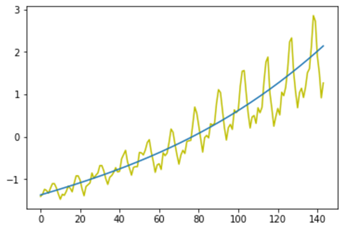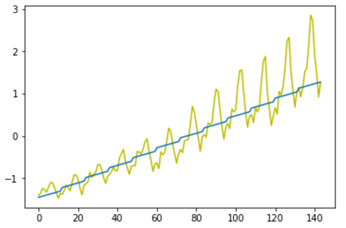上述程序都基于线性拟合，由于月份和乘客量并无单调上升关系（年峰值在七八月），因此，线性拟合方法效果也不是很好。读者如有兴趣，可以通过预处理进一步优化该程序的效果。
总结
本篇介绍的方法，使用Pytorch深度学习框架解决了线性回归问题，第一个例程中实现的是一元线性回归，第二个例程使用的方法类似于SVM中的核函数以及多元线性回归。
使用机器学习或者传统的统计学方法也能实现线性回归，且一些机器学习方法能更好地拟合本例中的曲线。本篇主要通过例程介绍全连接层的功能和用法，只使用了一层，还谈不上深度学习。在下篇中将使用RNN网络拟合本例中的数据，以达到更好的拟合效果，并借此介绍循环网络的原理、具体实现、以及RNN相关API的调用方法。
展开全文• 本文章讲解以拟合为设计目的的全连接神经网络模型，神经网络即可进行线性回归，也可进行非线性回归，并且是还是分段拟合，线性与非线性取决于激活函数！ 先来简单的一元二次函数： 看代码： x = torch.unsqueeze...
神经网络可作为一个函数，例如x为训练样本矩阵，f为神经网络，则f(x)会得到一个输出，输出表示啥取决于训练目的和训练过程。 本文章讲解以拟合为设计目的的全连接神经网络模型，神经网络即可进行线性回归，也可进行非线性回归，并且是还是分段拟合，线性与非线性取决于激活函数！
先来简单的一元二次函数： 看代码： x = torch.unsqueeze(torch.linspace(-1,1,100),dim=1) y = x.pow(2)+0.2*torch.rand(x.size()) plt.scatter(x.data.numpy(),y.data.numpy()) plt.show() 其图像为：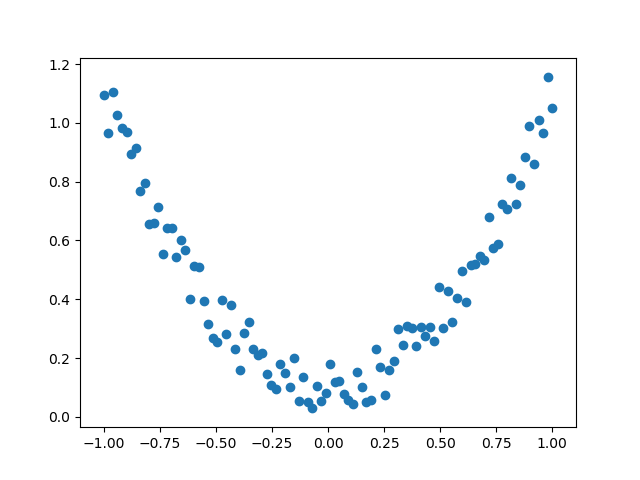采用线性激活函数版本： import torch import torch.nn.functional as F#激活函数都在这里 import matplotlib.pyplot as plt
#假数据：y = a*x^2+b,增加一些噪点，显得更加真实
x = torch.unsqueeze(torch.linspace(-1,1,100),dim=1)
y = x.pow(2)+0.2*torch.rand(x.size())
#画图
plt.scatter(x.data.numpy(),y.data.numpy())
plt.show()
class Net(torch.nn.Module): def init(self,n_featuer,n_hidden1,n_hidden2,n_output): super(Net,self).init() #定义每层的样式 self.hidden1 = torch.nn.Linear(n_featuer,n_hidden1)#隐藏层的线性输出 self.hidden2 = torch.nn.Linear(n_hidden1, n_hidden2) # 隐藏层的线性输出 self.predict = torch.nn.Linear(n_hidden2,n_output)#输出层的线性输出 def forward(self, x): x = F.relu(self.hidden1(x)) x = F.relu(self.hidden2(x)) x = (self.predict(x)) return x
net = Net(1,10,10,1)
opt = torch.optim.SGD(net.parameters(),lr=0.2) loss_func = torch.nn.MSELoss()
plt.ion()
for t in range(500): out = net(x) loss = loss_func(out,y) opt.zero_grad() loss.backward() opt.step()
if t%5 == 0:
print(t)
plt.cla()
plt.scatter(x.data.numpy(),y.data.numpy())
plt.plot(x.data.numpy(),out.data.numpy(),'r-',lw=5)
plt.text(0.5,0,"Loss=%.4f"%loss.data.numpy(),fontdict={"size":20,"color":"red"})
plt.pause(0.1)

plt.ioff() plt.show() 效果：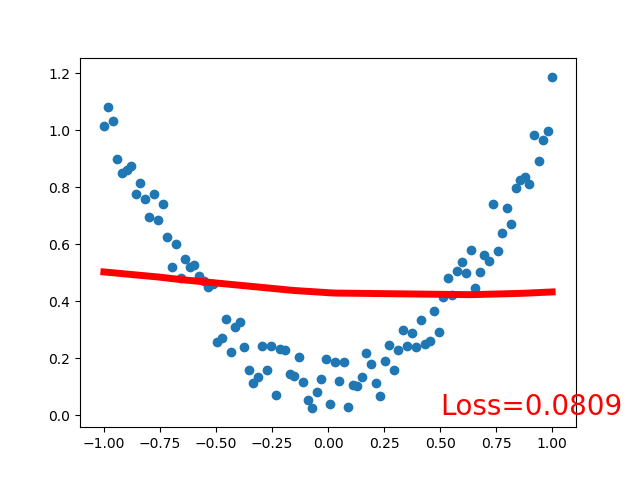注意：因为采用的线性激活函数，拟合的回归函数也是线性的，且是分段拟合
再看激活函数采用非线性的效果： 代码： import torch import torch.nn.functional as F#激活函数都在这里 import matplotlib.pyplot as plt
#假数据：y = a*x^2+b,增加一些噪点，显得更加真实
x = torch.unsqueeze(torch.linspace(-1,1,100),dim=1)
y = x.pow(2)+0.2*torch.rand(x.size())
#画图
plt.scatter(x.data.numpy(),y.data.numpy())
plt.show()
class Net(torch.nn.Module): def init(self,n_featuer,n_hidden1,n_hidden2,n_output): super(Net,self).init() #定义每层的样式 self.hidden1 = torch.nn.Linear(n_featuer,n_hidden1)#隐藏层的线性输出 self.hidden2 = torch.nn.Linear(n_hidden1, n_hidden2) # 隐藏层的线性输出 self.predict = torch.nn.Linear(n_hidden2,n_output)#输出层的线性输出 def forward(self, x): x = F.tanh(self.hidden1(x)) x = F.tanh(self.hidden2(x)) x = (self.predict(x)) return x
net = Net(1,10,10,1)
opt = torch.optim.SGD(net.parameters(),lr=0.2) loss_func = torch.nn.MSELoss()
plt.ion()
for t in range(500): out = net(x) loss = loss_func(out,y) opt.zero_grad() loss.backward() opt.step()
if t%5 == 0:
print(t)
plt.cla()
plt.scatter(x.data.numpy(),y.data.numpy())
plt.plot(x.data.numpy(),out.data.numpy(),'r-',lw=5)
plt.text(0.5,0,"Loss=%.4f"%loss.data.numpy(),fontdict={"size":20,"color":"red"})
plt.pause(0.1)

plt.ioff() plt.show() 效果：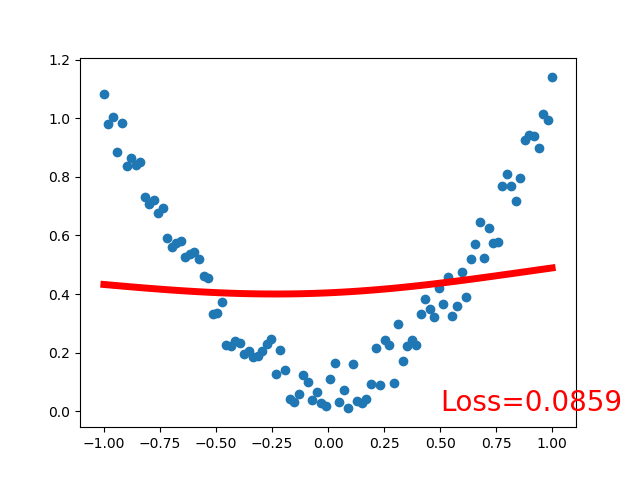可见，采用线性激活函数和非线性激活函数的区别很大，得看具体应用场景，激活函数关系到训练效果，激活函数不恰当会导致模型训练不到理想的效果
展开全文神经网络 回归
• 为了方便开发者应用，PyTorch专门开发了一个视觉工具包torchvision，主要包含以下三个部分： 1.model机器学习 神经网络 深度学习
• import torch import torchvision from torchvision import transforms from torch import nn from torch.autograd import Variable import torch.nn.functional as F import matplotlib.pyplot as plt ...
• import numpy as np import torch from torchvision.datasets import mnist from torch import nn from torch.autograd import Variablepython
• Pytorch中已经封装好了组成全连接神经网络的部件 ，即线性层与非线性激活层，如果模型只是单纯的线性层叠加，最后模型也是线性的，等价于只有一个线性层，加入非线性激活层才使得深度有了意义。 ...非线性激活函数 深度学习 单层感知机
• * correct / len(test_loader.dataset))) 输出结果： runfile('E:/workspace/pytorch-learn/27_MLP网络层/main.py', wdir='E:/workspace/pytorch-learn/27_MLP网络层') Train Epoch: 0 [0/60000 (0%)] Loss: 2....
• pd.crosstab(train_data['Title'], train_data['Survived'])python...